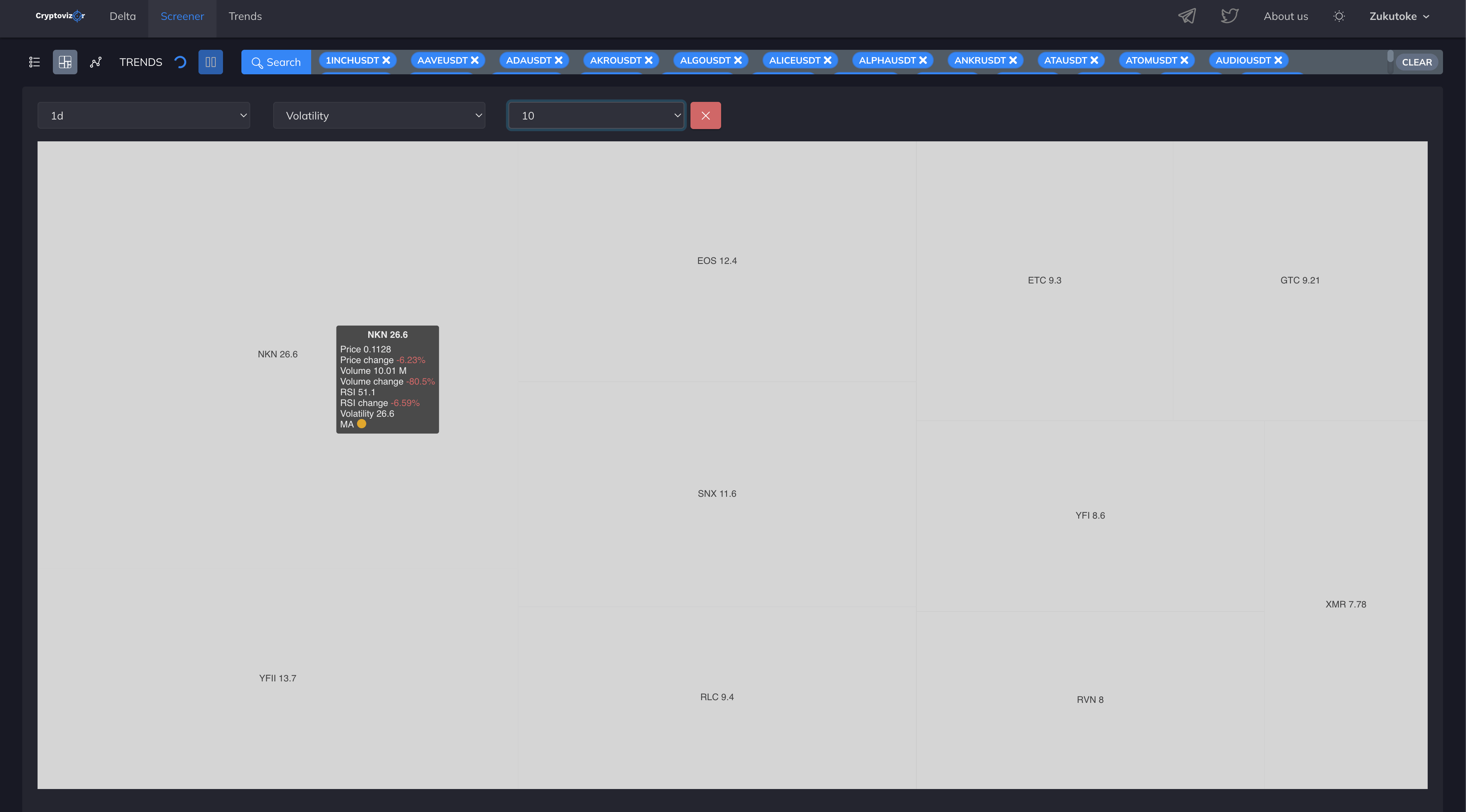# Cryptocurrency heatmap Binance

## Heatmap module

A heat map is a visualized space divided into different rectangles. The size and order of these rectangles is determined by a quantitative variable.

Such a variable can be the following columns of the screener table:

Volume – trading volume in USDT

Volume change – volume change percentage

Price change – price change percentage

RSI change – percentage of change in the RSI indicator value

Volatility – price volatility value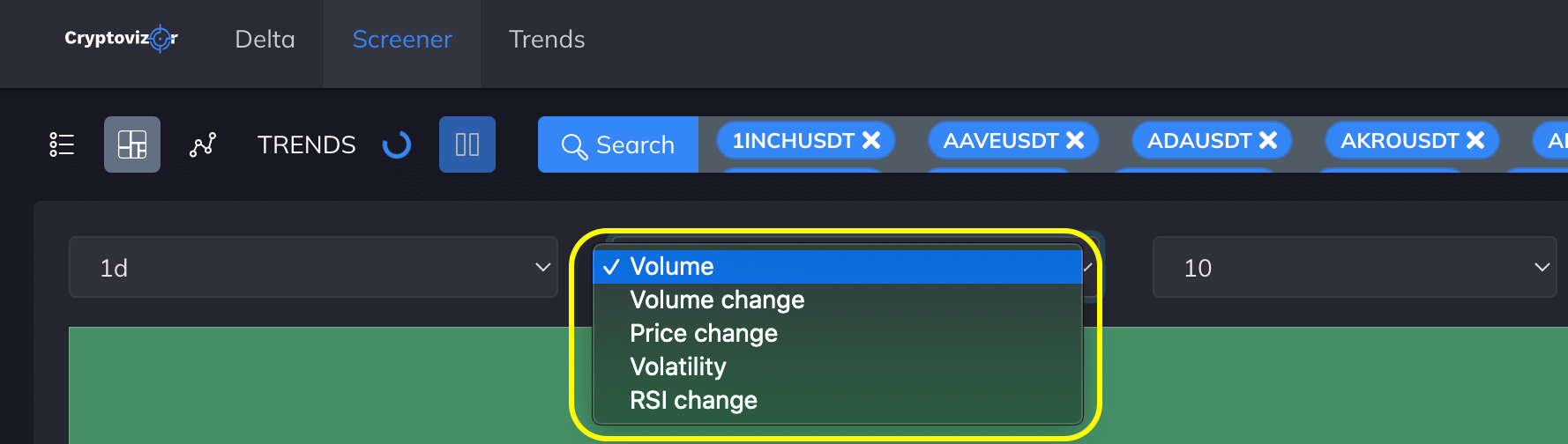Each of the selected filters operates in all timeframes: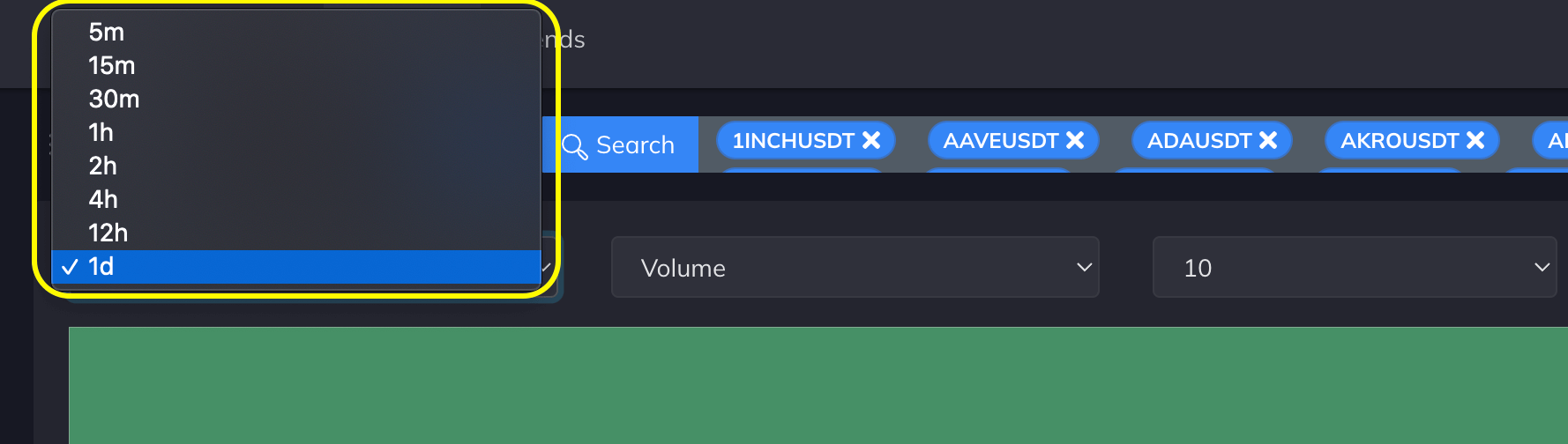For more convenient work, you can choose the maximum number of displayed elements in the heat map – from ten to three hundred.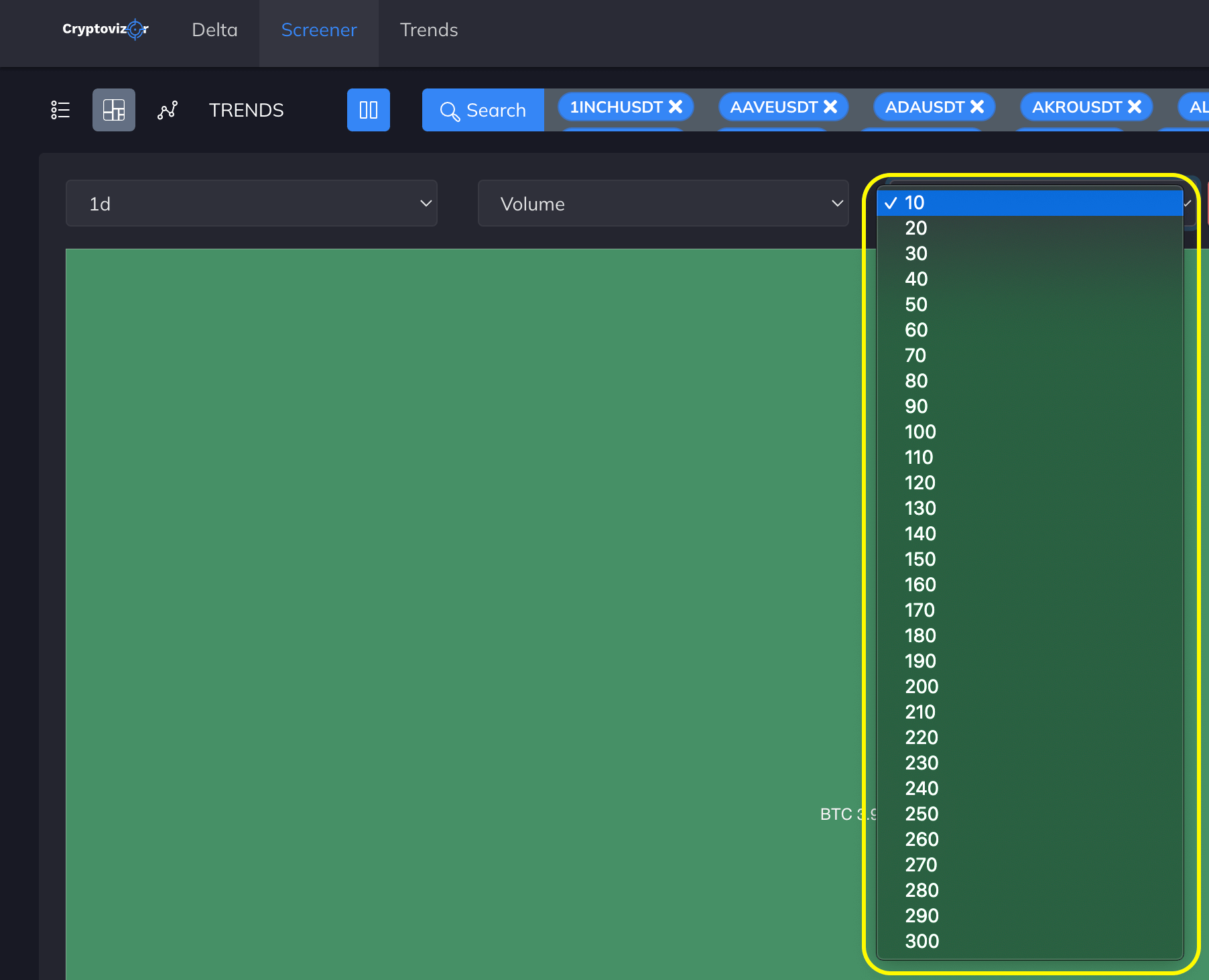### What coins are involved in building a heat map?

In the event that nothing is selected in the search bar, then all USDT coin pairs on Binance Spot participate.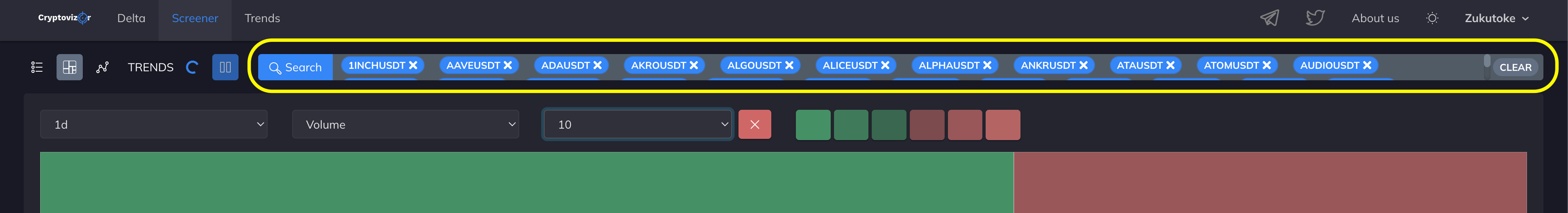Best practices – using a heat map based on the watchlist or on the coins that are selected in the search bar.

Let's take a closer look at each filter below:

### Volume

Volume” is the trading volume in USDT. As you know, we use a moving method of trading volume calculation. It shows the value of the current moment minus N periods ago, where N is the selected timeframe

The moving and per-bar trading volumes differ in that when the candle closes, the volume value is not reset to zero.

If you select the Volume filter with a 1D timeframe and ten displayed elements, the map will display the top ten coins by trading volume from the watchlist or list of coins you selected. If the search field is left empty, the map will be generated based on all the coins from the screener.

The size of each cell will indicate the ratio of the maximum value of the selected filter relative to the minimum value.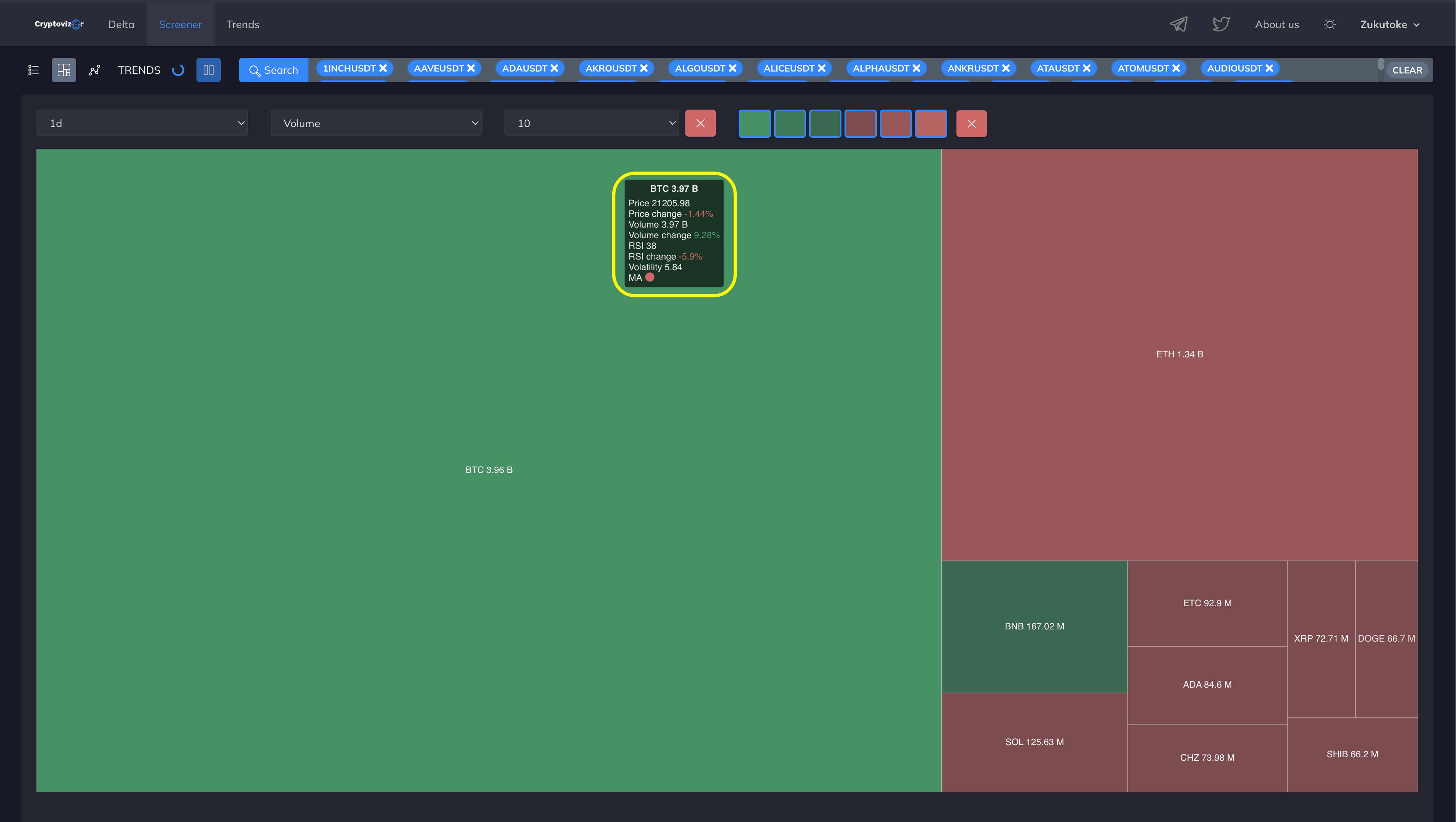If you hover over a cell of the map, you will see the values ​​for the current timeframe in a pop-up window.

TICKER

Price – current price

Price change – price change percentage

Volume – trading volume in USDT

Volume change – volume change percentage

RSI – the value of the RSI indicator with a standard period of 14

RSI change – percentage change in the value of the RSI indicator

Volatility – price volatility value

MA – the value of the state of the trend by moving averages. (The default is MA50 and MA200. You can set other values ​​in the settings).

The color in which the cell will be displayed will depend on the value of Volume change (the percentage change in trading volume). If the volume changes down, the cell will be red, if it has increased, it will turn green.

And the brightness of the cell directly depends on the value of Volume (trading volume). Light green or red indicates the highest trading volume, while dark color indicates the lowest trading volume among the selected coins.

If you click on a cell of the map, a chart will open in the selected timeframe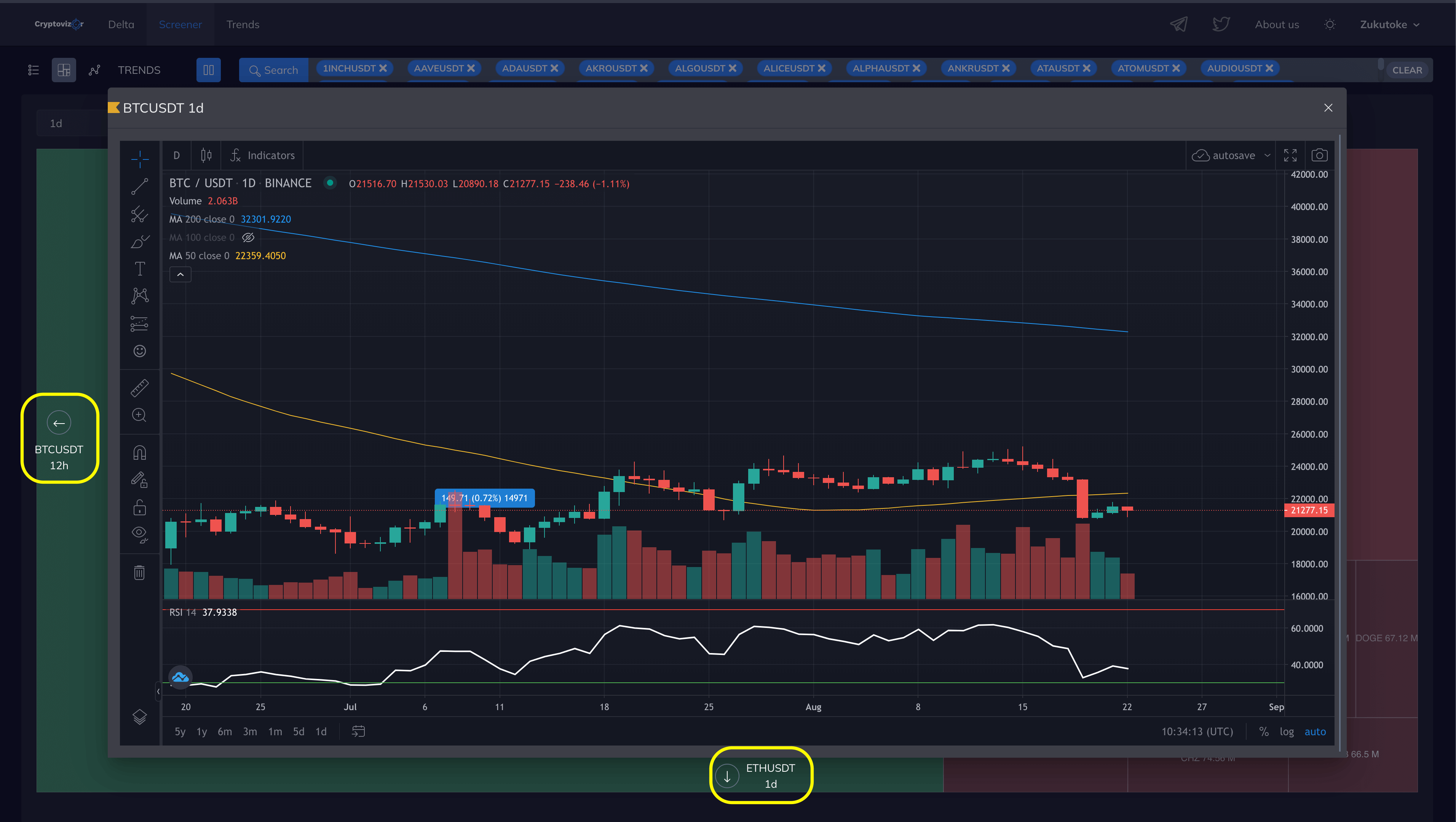To make navigation more convenient and to switch timeframes, you can use the buttons on the borders of the chart window or the arrows on your keyboard.

We have combined the markup on the charts with all screener tabs. You can start working with the heatmap and then continue with the markup in the table. Everything will be saved automatically.

### Volume change

Volume change is the percentage change in trading volume.

As already mentioned, we use a moving method for calculating the volume of trades. It shows the value of the current moment minus N periods ago, where N is the selected timeframe

The moving and per-bar trading volumes differ in that when the candle closes, the volume value is not reset to zero.

If the 1D timeframe is selected, the Volume change filter and the maximum number of displayed elements in the heatmap is 10, then you will see the top 10 coins by the percentage of volume change per 1D (24H). In this case, the beginning of the period (Y) 1D will be the current moment (X) minus 24 hours (N)

Y=X–N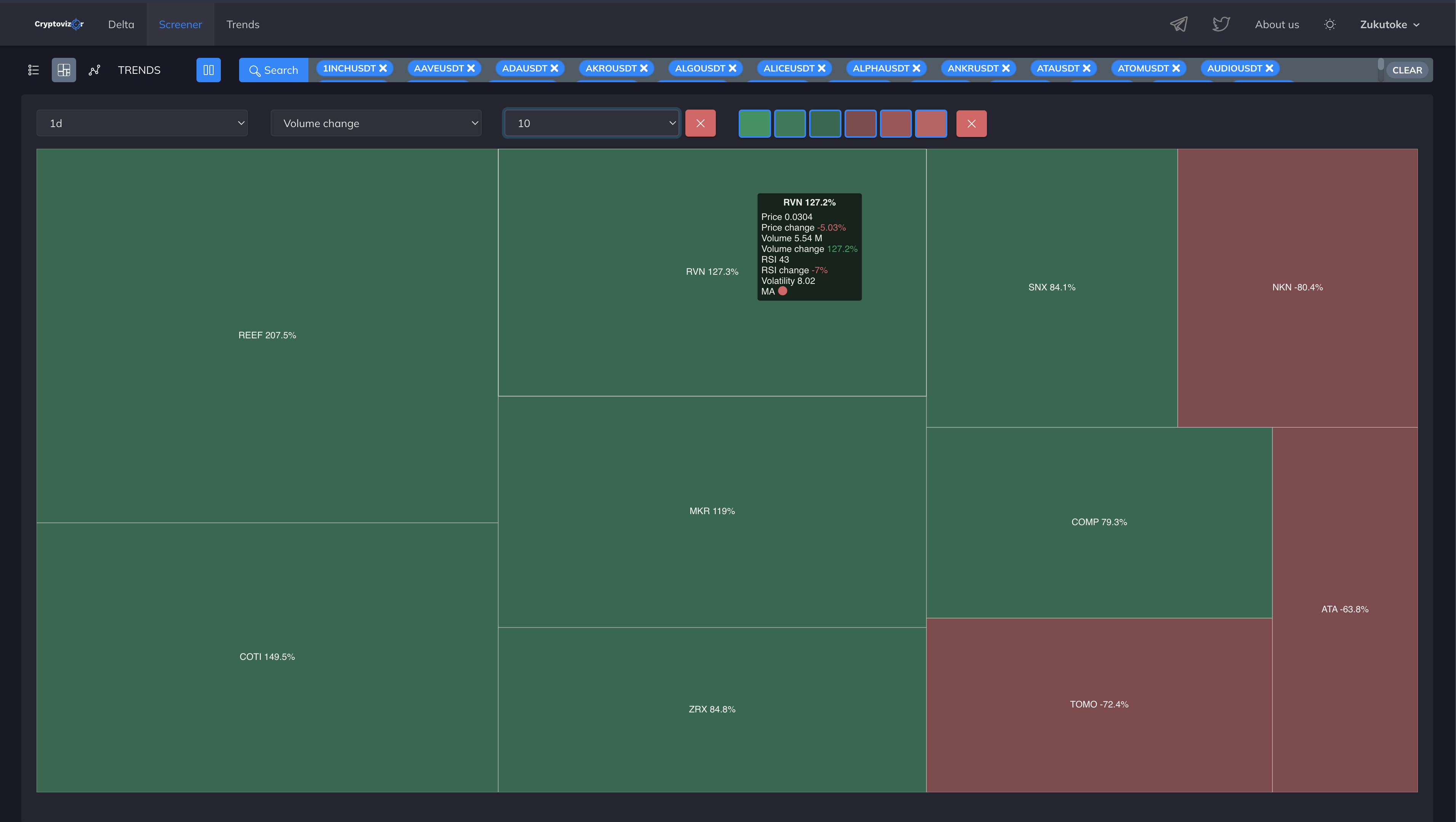The color of the cell will depend on the value of Volume change (the percentage change in trading volume). If the volume changes down, the cell will be red, if it has increased, it will turn green.

And the brightness of the cell directly depends on the value of Volume (trading volume). Light green or red indicates the highest trading volume, while dark color indicates the lowest trading volume among the selected coins.

### Price change

Price change is a filter for tracking the percentage of price change.

Here the cell color will depend on the Price change value. If the percentage of price change becomes negative, then the fill will turn red, if positive, it will turn green.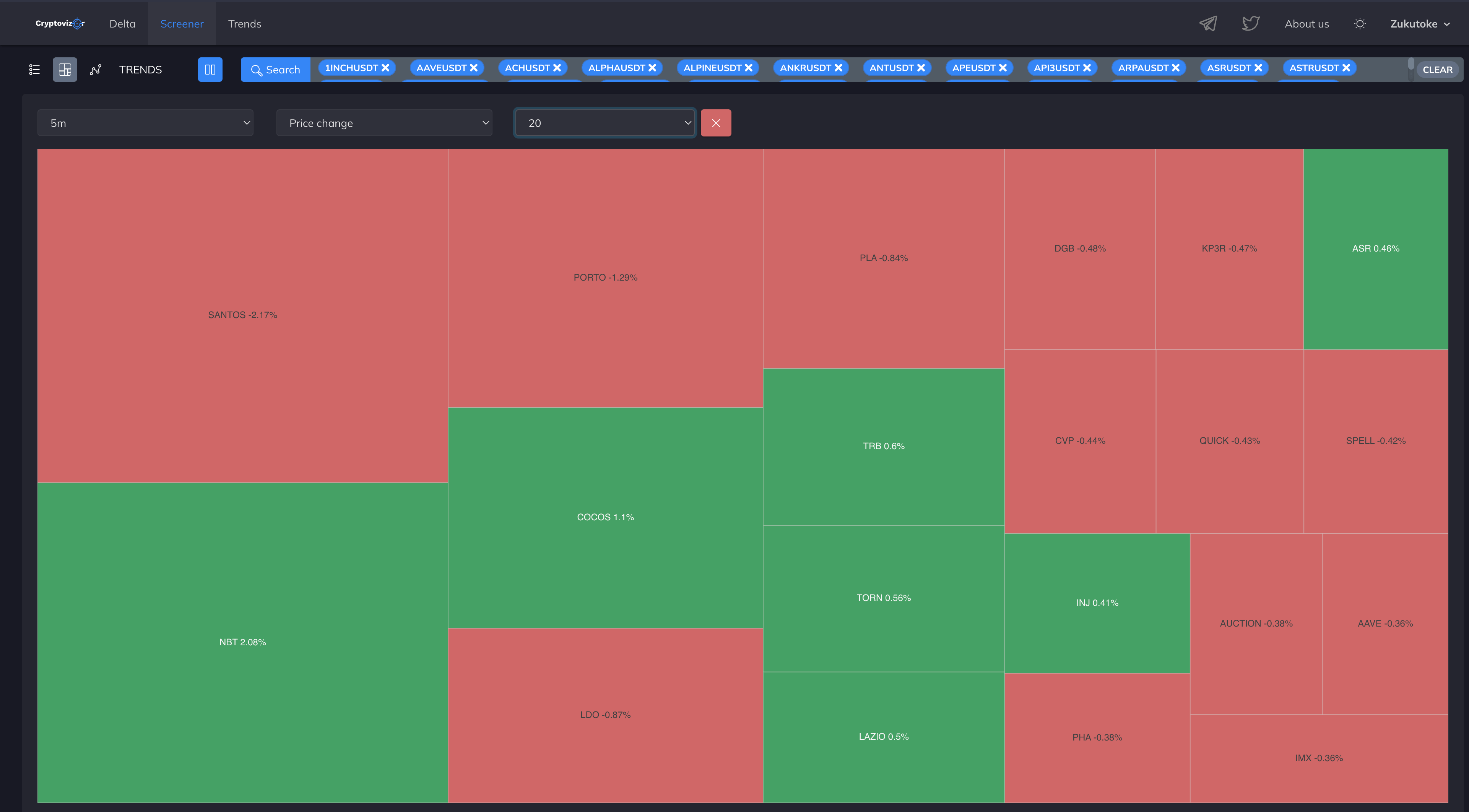### RSI change

RSI change is a filter for tracking the percentage change in RSI indicator values.

The color of the cell will depend on the RSI change value. If the percentage of price change becomes negative, then the fill will turn red, if positive, it will turn green.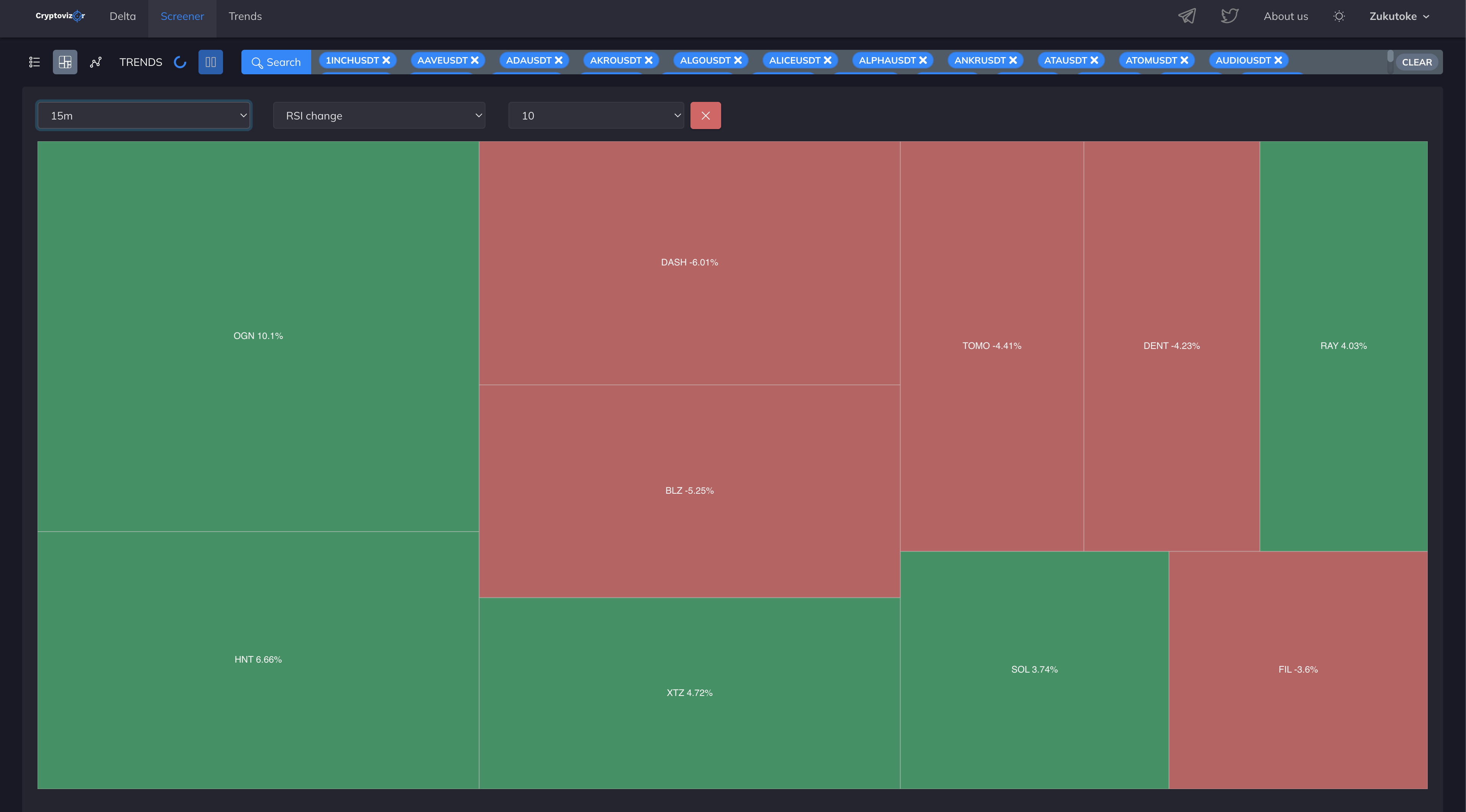### Volatility

Volatility is a price volatility tracking filter. The higher the value that is displayed, the higher the volatility is, and vice versa.